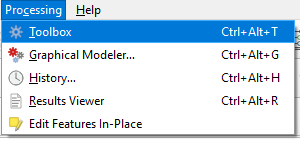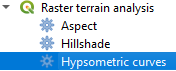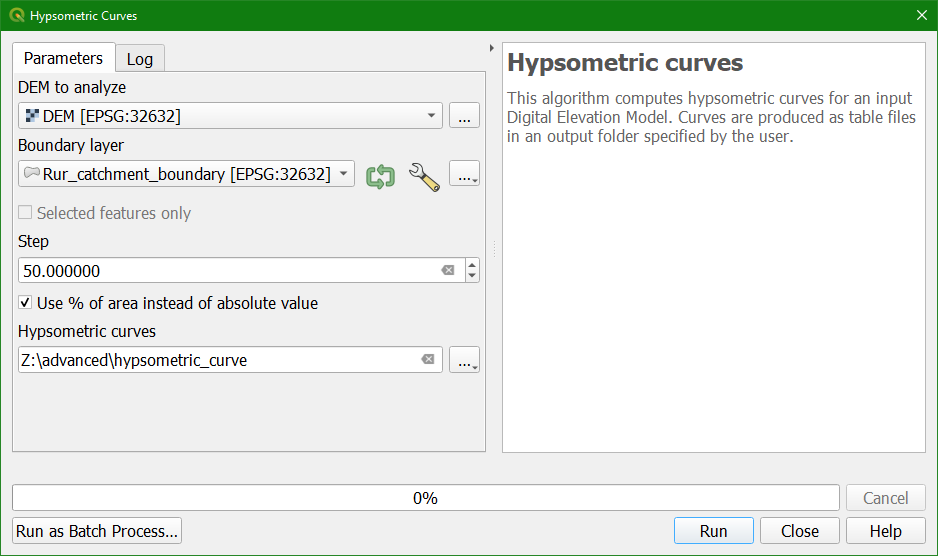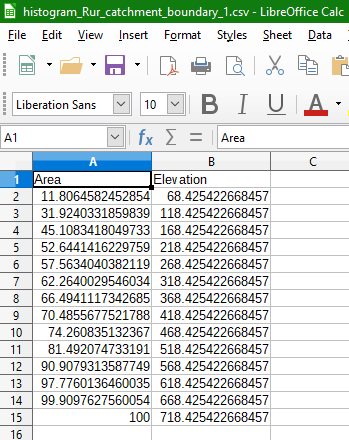## Tutorial: Create a Hypsometric Curve

This tutorial explains how to create a hypsometric curve in QGIS

### 3. Create the cumulative elevation distribution table

In this section we're going to calculate the cumulative elevation distribution table.

1. Open the Processing Toolbox: from the main menu choose Processing | Toolbox.2. In the Processing Toolbox choose Raster terrain analysis | Hypsometric curves3. In the Hypsometric Curves dialogue choose the DEM as DEM to analyze and Rur_catchment_boundary as the Boundary layer. The algorithm will only consider the elevation within this boundary layer. Change the Step to 50 meters. That is the elevation interval for which the cumulative percentages of the area will be calculated. To get the percentages check the box to Use % of area instead of absolute value. Choose an output folder to save the CSV (comma separated values) file and click Run.4. Click Close when the algorithm has finished.

5. Go to the folder with and check if it has created histogram_Rur_catchment_boundary_1.csv. Inspect the file in a spreadsheet programme, such as LibreOffice Calc.There you can see in column A the areas in cumulative percentage of the total catchment area and in column B the elevations. So this means that 11.8% of the catchment has an elevation <= 68.5 m and 100% of the catchment has an elevation <= 718.4 m.

In the next section we'll create the curve from this table using the DataPlotly plugin.